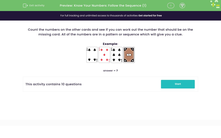# Follow a Sequence of Numbers to Find the Missing Number

In this worksheet, students will use their backwards and forwards counting skills to identify the missing number.Key stage:  KS 1

Curriculum topic:   Number: Number and Place Value

Curriculum subtopic:   Count to 100

Difficulty level:#### Worksheet Overview

In this activity, we will be working out the missing number in a sequence of numbers up to 100.

We can use the number square to help us if it is tricky.There are two steps to solve each question.

First, we need to work out if it is going forwards or backwards.

Then, we need to count along and work out which number is missing. We can use the number square if it is a bit tricky.

Example

12, 13, 14, 15,  _ , 17

Is this number pattern going forwards or backwards? The numbers are getting bigger, so this means it is going forwards.

Now, let's count along from 12 and work out what the missing number is. To help, we can look for 12 on the number square and count along with our finger until we get to the missing number:The missing number is after 15, so it is 16.

Shall we try some questions now?### What is EdPlace?

We're your National Curriculum aligned online education content provider helping each child succeed in English, maths and science from year 1 to GCSE. With an EdPlace account you’ll be able to track and measure progress, helping each child achieve their best. We build confidence and attainment by personalising each child’s learning at a level that suits them.

Get started